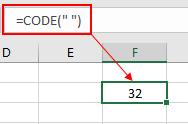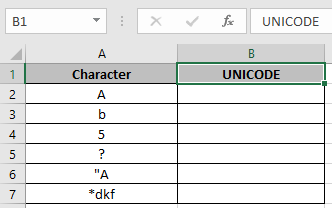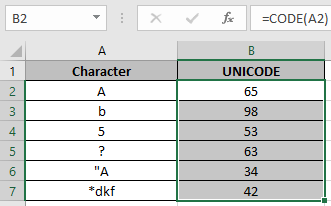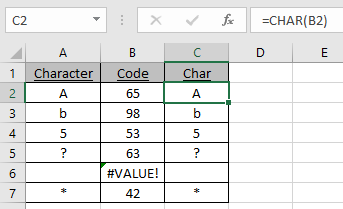# UNICODE function

In this article, we will learn about how to use the UNICODE function in Excel.
Unicode is computing standard for the unified encoding, representation, and handling of text in most of the world's writing systems. The text value used to retrieve the code character. Only the first character of the text value will be converted to a Unicode number.
The UNICODE function returns a numerical Unique code of any character in Excel.
Syntax:

=UNICODE ( “ text ” )

Text : in quotes or using the cell reference.
Let’s understand this function using it in an example.As you can see in the above snapshot the UNICODE function takes the character single space in quotation marks(“ ”) as an argument and returns its defined numeric unique code for the single space which is 32.

Now we will understand it using cell references in the formula.

Here we have a list of characters we need the UNICODE of
Characters using the function.Use the formula:

=UNICODE ( A2 )

A2 : argument provided as cell reference but provides the code for the first char in the referenced cell.Here the argument to the function is given as cell reference.
Copy the formula in other cells using shortcut Ctrl + D.The function returns a Value error if empty cell is given as an argument.

You can use the UNICHAR function to get the character out of a UNICODE.As you can see the UNICODE function returns the ANSI CODE whereas UNICHAR function returns the character from the CODE.

Notes:

1. The function returns #VALUE! Error, If the text is invalid, as excel have predefined text format.
2.  Use the UNICHAR function to perform the just exact opposite to UNICODE function. UNICHAR function returns the char for the given input code to the function.
3. Text argument to the function can be given as cell reference and directly using the quotation marks.

Hope you understood how to use the UNICODE function and referring cell in Excel. Explore more articles on Excel TEXT functions here. Please feel free to state your query or feedback for the above article.
Related Articles

How to use the CODE function in Excel

How to use the CHAR function in Excel

How to use Wildcards in Excel

Popular Articles

Edit a dropdown list

If with conditional formatting

If with wildcards

Vlookup by date

Terms and Conditions of use

The applications/code on this site are distributed as is and without warranties or liability. In no event shall the owner of the copyrights, or the authors of the applications/code be liable for any loss of profit, any problems or any damage resulting from the use or evaluation of the applications/code.